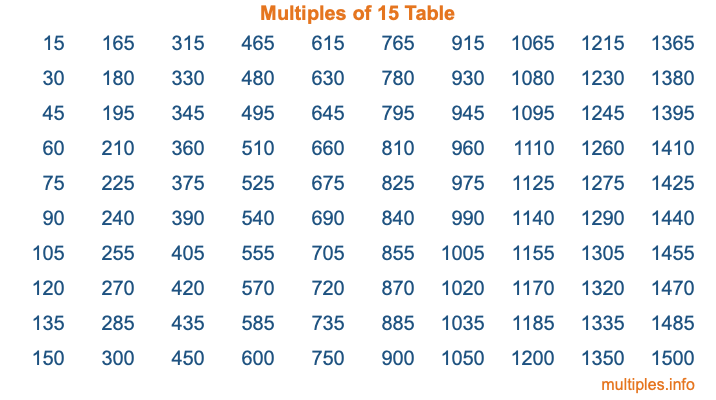Multiples of 15Welcome to the Multiples of 15 page. Here we will first teach you everything you will ever need to know about the multiples of 15, and then give you a study guide summary of everything we taught you to make sure you remember it all. Use this page to look up facts and learn information about the multiples of 15. This page will make you a multiples of fifteen expert!

Definition of Multiples of 15
Multiples of 15 are all the numbers that when divided by 15 equal an integer. Each of the multiples of 15 are called a multiple. A multiple of 15 is created by multiplying 15 by an integer.

Therefore, to create a list of multiples of 15, you start with 1 multiplied by 15, then 2 multiplied by 15, then 3 multiplied by 15, and so on for as long as you want. Thus, the list of the first five multiples of 15 is 15, 30, 45, 60, and 75. To see a larger list of multiples of 15, see the printable image of Multiples of 15 further down on this page. We also have a category where you can choose any nth multiple of 15.

Multiples of 15 Checker
The Multiples of 15 Checker below checks to see if any number of your choice is a multiple of 15. In other words, it checks to see if there is any number (integer) that when multiplied by 15 will equal your number. To do that, we divide your number by 15. If the the quotient is an integer, then your number is a multiple of 15.

Is  a multiple of 15?

Least Common Multiple of 15 and ...
A Least Common Multiple (LCM) is the lowest multiple that two or more numbers have in common. This is also called the smallest common multiple or lowest common multiple and is useful to know when you are adding our subtracting fractions. Enter one or more numbers below (15 is already entered) to find the LCM.

Check out our LCM Calculator if you need more details about the Least Common Multiple or if you need the LCM for different numbers for adding and subtraction fractions.

nth Multiple of 15
As we stated above, 15 is the first multiple of 15, 30 is the second multiple of 15, 45 is the third multiple of 15, and so on. Enter a number below to find the nth multiple of 15.

th multiple of 15

Multiples of 15 vs Factors of 15
15 is a multiple of 15 and a factor of 15, but that is where the similarities end. All postive multiples of 15 are 15 or greater than 15. All positive factors of 15 are 15 or less than 15.

Below is the beginning list of multiples of 15 and the factors of 15 so you can compare:

Multiples of 15: 15, 30, 45, 60, 75, etc.

Factors of 15: 1, 3, 5, 15

As you can see, the multiples of 15 are all the numbers that you can divide by 15 to get a whole number. The factors of 15, on the other hand, are all the whole numbers that you can multiply by another whole number to get 15.

It's also interesting to note that if a number (x) is a factor of 15, then 15 will also be a multiple of that number (x).

Multiples of 15 vs Divisors of 15
The divisors of 15 are all the integers that 15 can be divided by evenly. Below is a list of the divisors of 15.

Divisors of 15: 1, 3, 5, 15

The interesting thing to note here is that if you take any multiple of 15 and divide it by a divisor of 15, you will see that the quotient is an integer.

Multiples of 15 Table
Below is an image of the first 100 multiples of 15 in a table. The table is in chronological order, column by column. The first column has the first ten multiples of 15, the second column has the next ten multiples of 15, and so on.The Multiples of 15 Table is also referred to as the 15 Times Table or Times Table of 15. You are welcome to print out our table for your studies.

Negative Multiples of 15
Although not often discussed or needed in math, it is worth mentioning that you can make a list of negative multiples of 15 by multiplying 15 by -1, then by -2, then by -3, and so on, to get the following list of negative multiples of 15:

-15, -30, -45, -60, -75, etc.

Multiples of 15 Summary
Below is a summary of important Multiples of 15 facts that we have discussed on this page. To retain the knowledge on this page, we recommend that you read through the summary and explain to yourself or a study partner why they hold true.

There are an infinite number of multiples of 15.

A multiple of 15 divided by 15 will equal a whole number.

15 divided by a factor of 15 equals a divisor of 15.

The nth multiple of 15 is n times 15.

The largest factor of 15 is equal to the first positive multiple of 15.

15 is a multiple of every factor of 15.

15 is a multiple of 15.

A multiple of 15 divided by a divisor of 15 equals an integer.

15 divided by a divisor of 15 equals a factor of 15.

Any integer times 15 will equal a multiple of 15.

Multiples of a Number
Here you can get the multiples of another number, all with the same attention to detail as we did for multiples of 15 on this page.

Multiples of
Multiples of 16
Did you find our page about multiples of fifteen educational? Do you want more knowledge? Check out the multiples of the next number on our list!